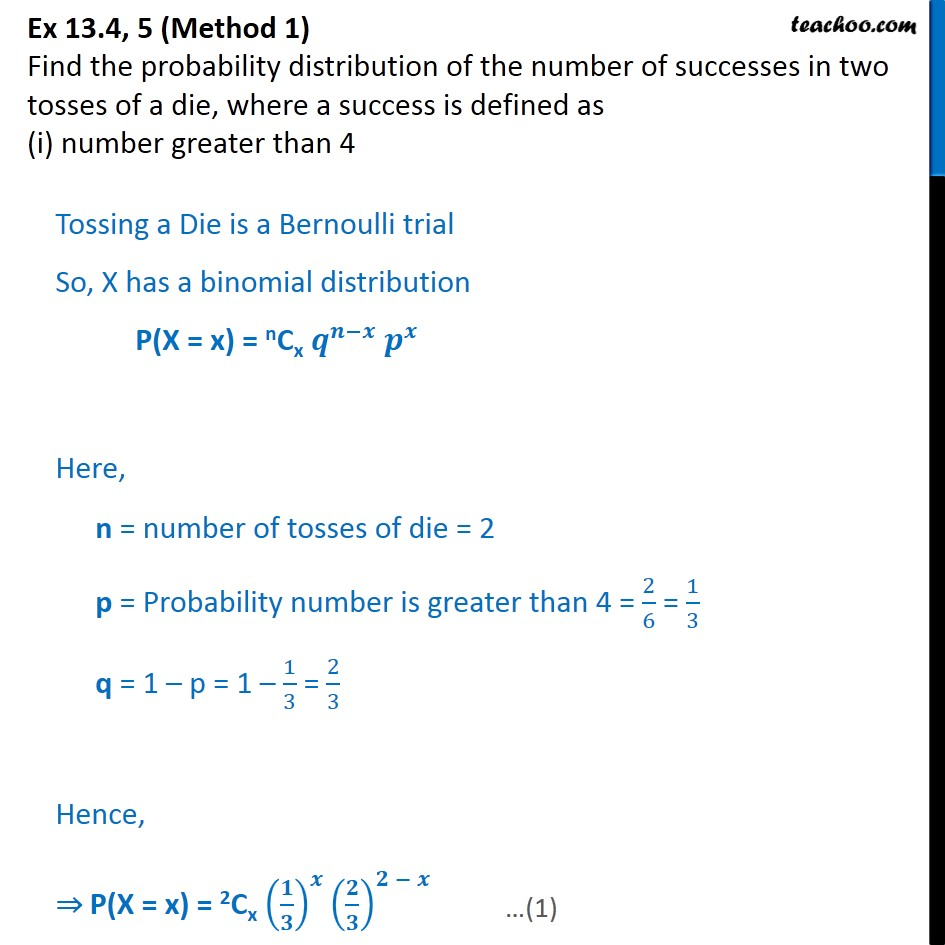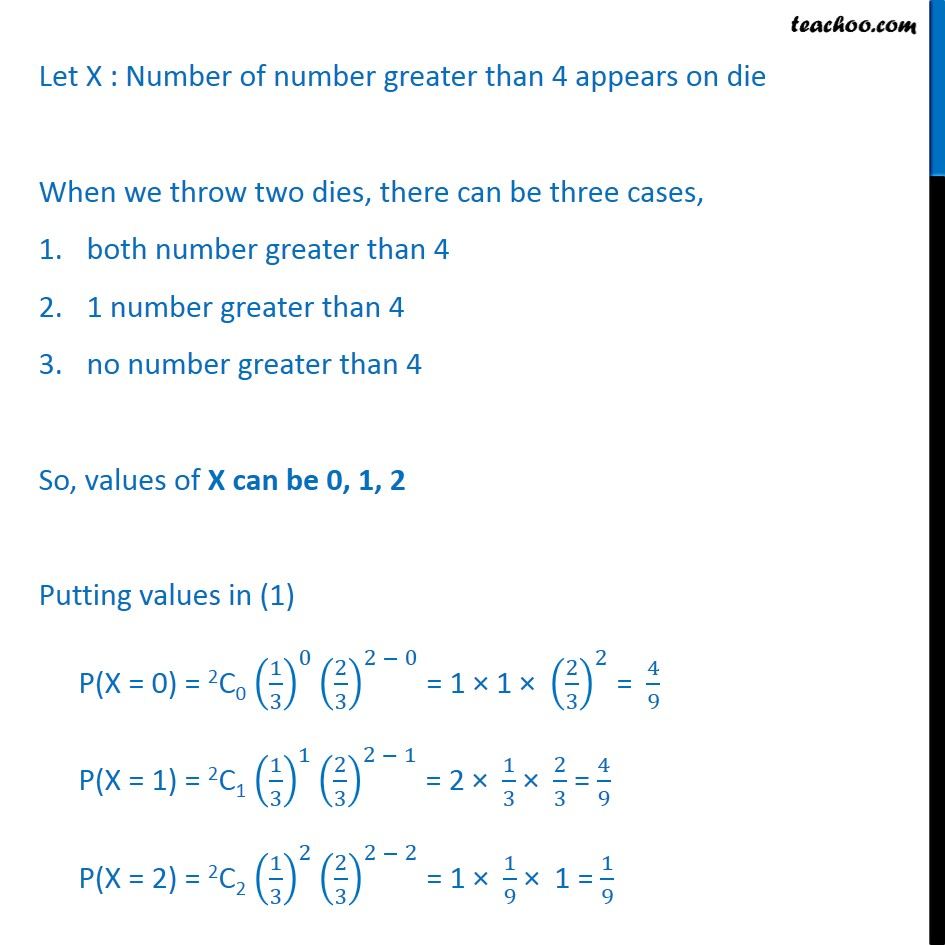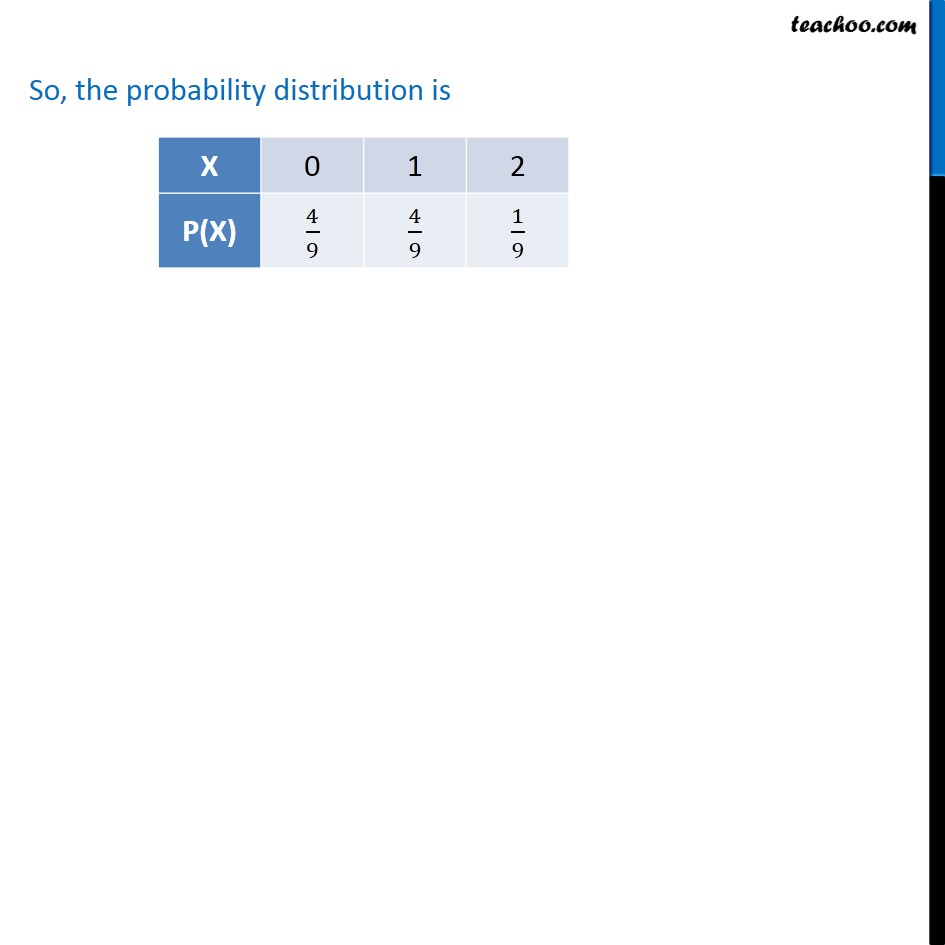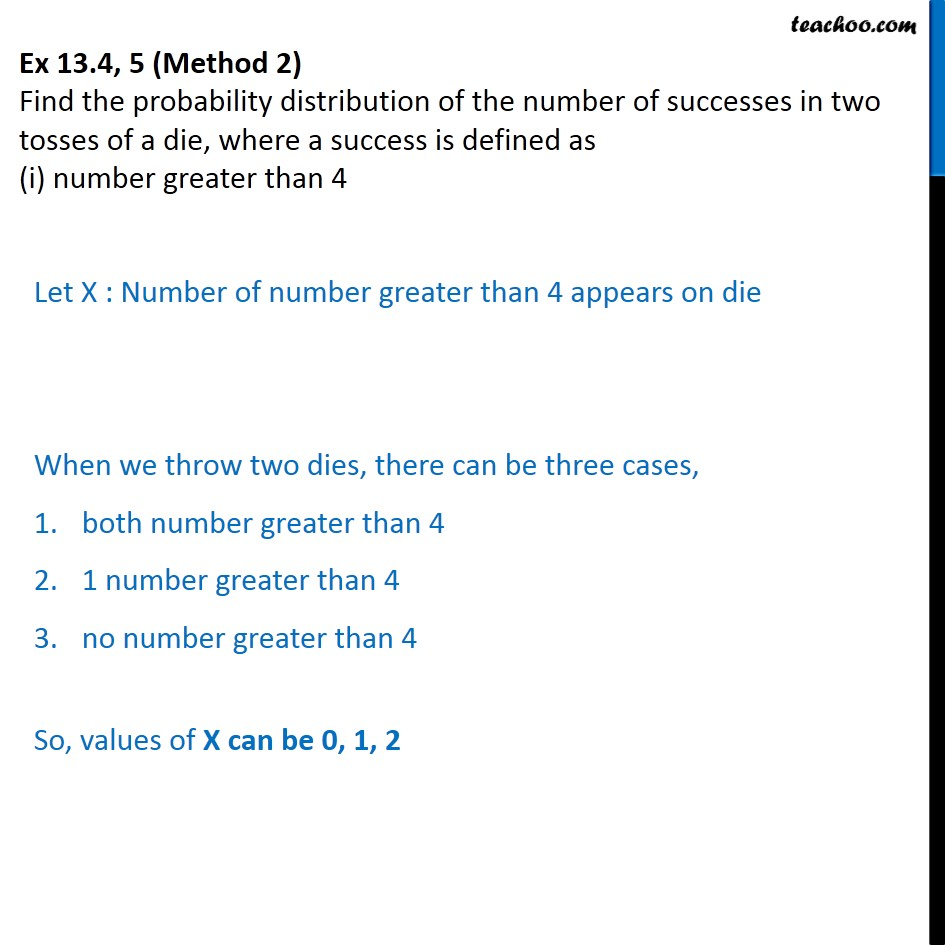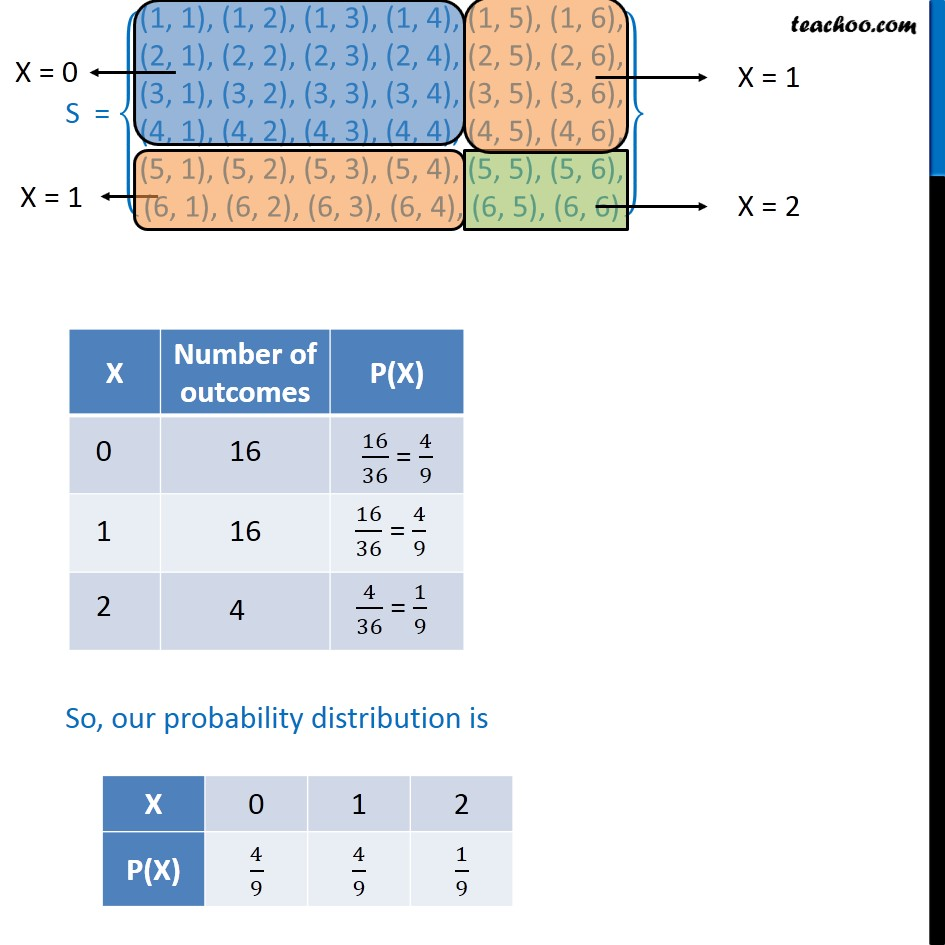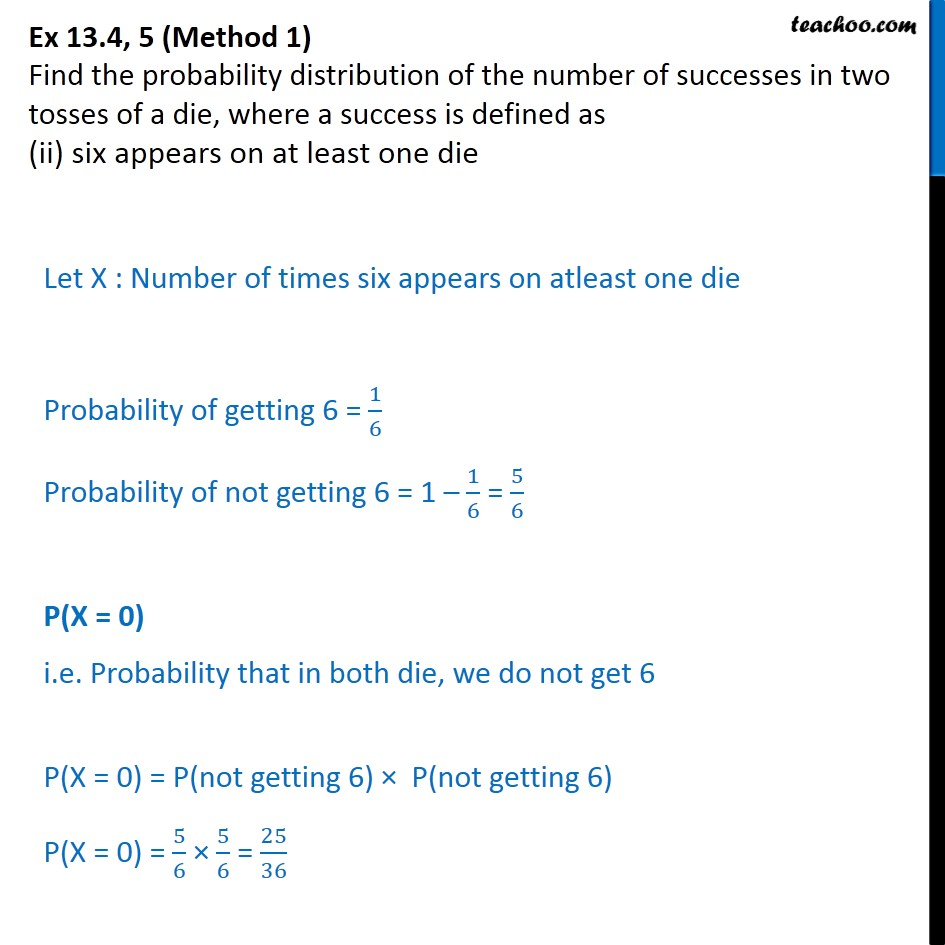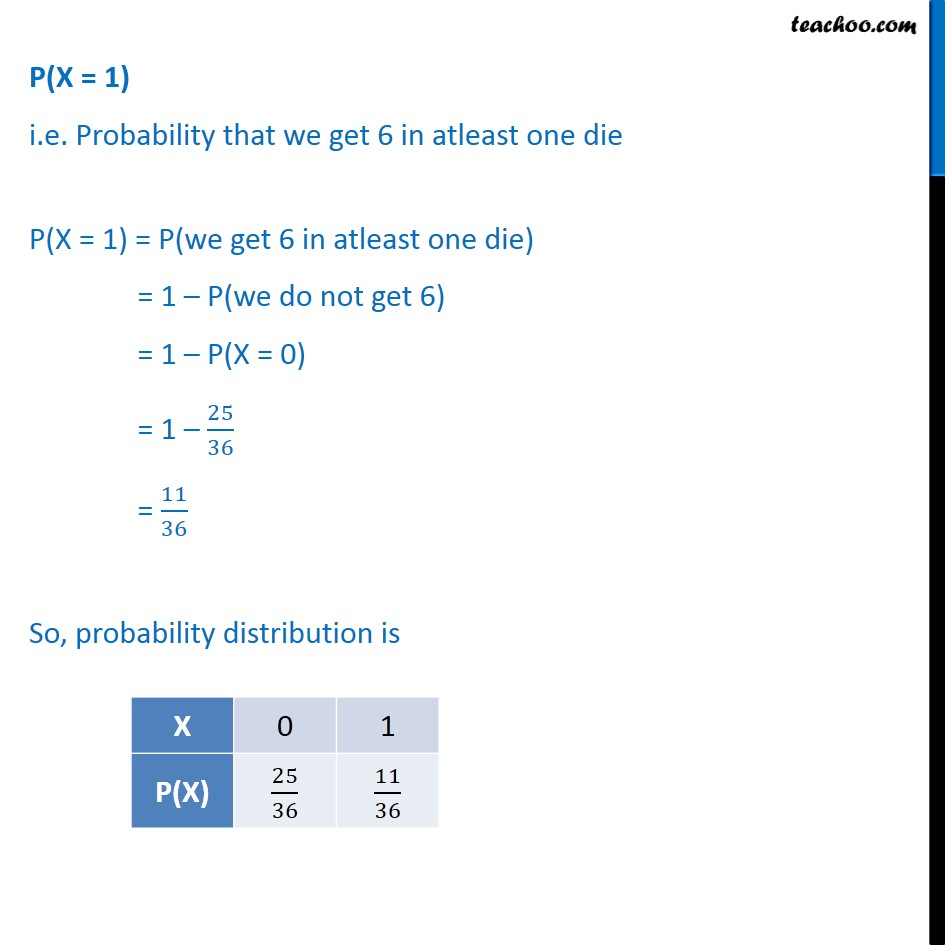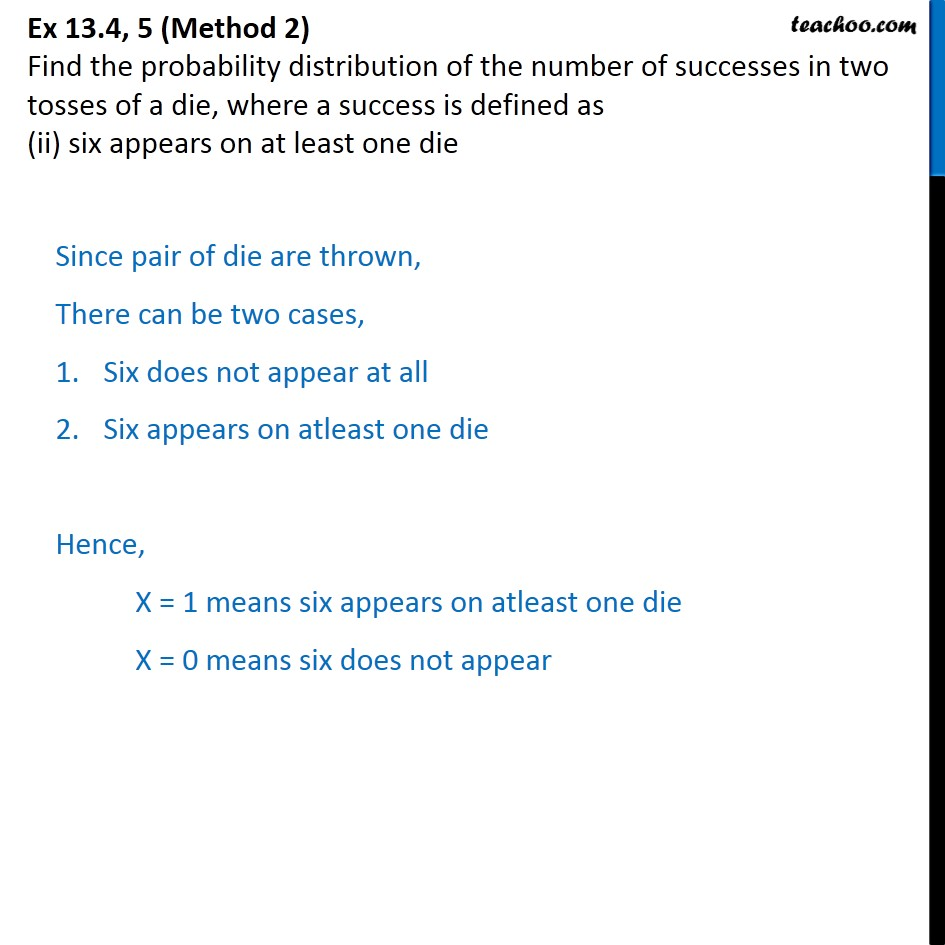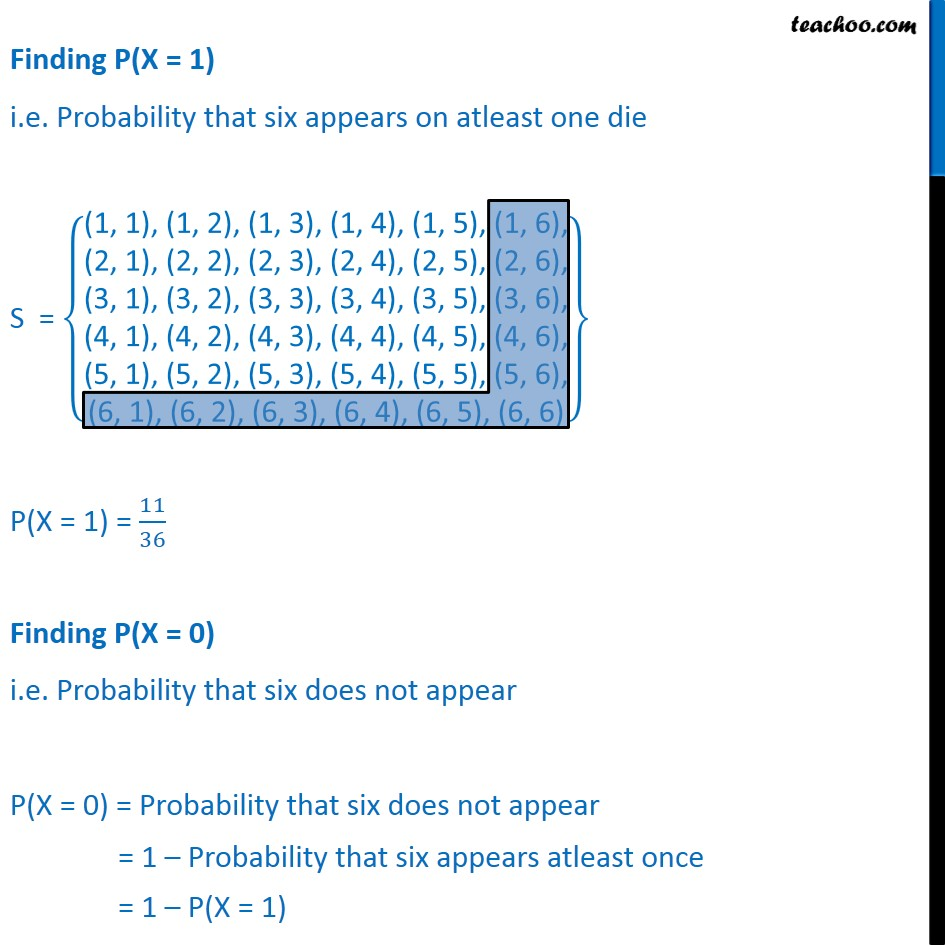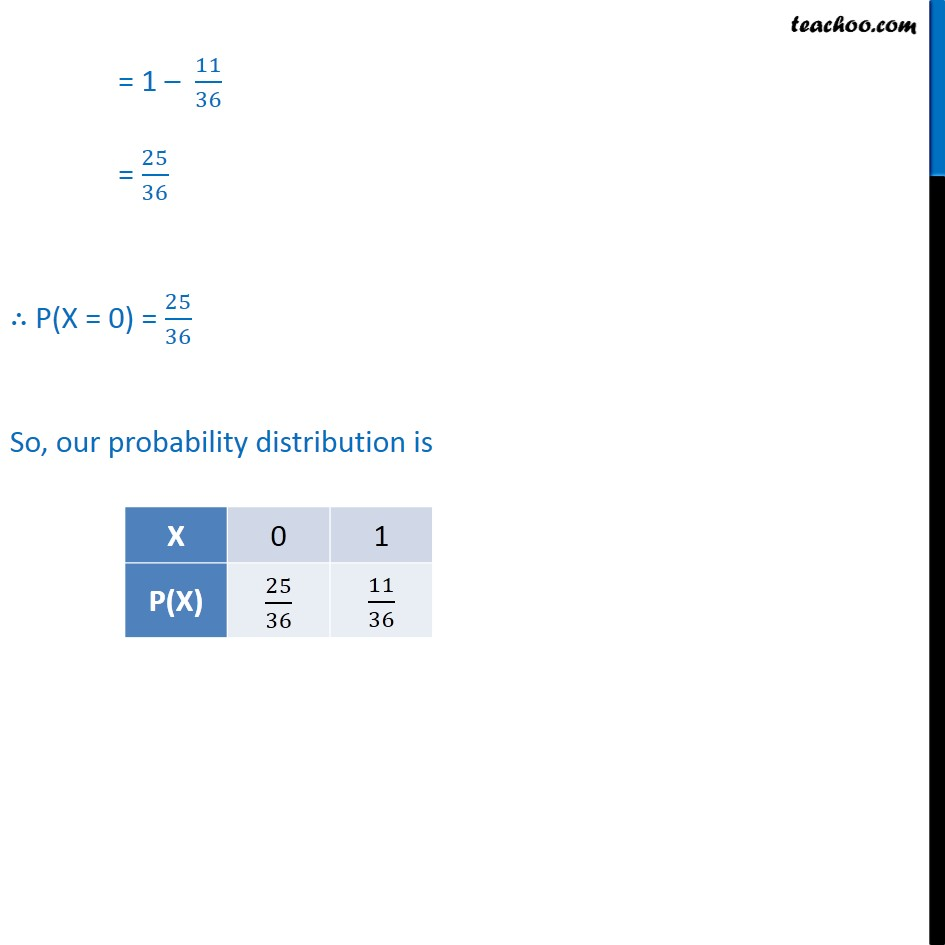1. Chapter 13 Class 12 Probability
2. Serial order wise
3. Ex 13.4

Transcript

Ex 13.4, 5 (Method 1) Find the probability distribution of the number of successes in two tosses of a die, where a success is defined as (i) number greater than 4 Tossing a Die is a Bernoulli trial So, X has a binomial distribution P(X = x) = nCx 𝒒﷮𝒏−𝒙﷯ 𝒑﷮𝒙﷯ Here, n = number of tosses of die = 2 p = Probability number is greater than 4 = 2﷮6﷯ = 1﷮3﷯ q = 1 – p = 1 – 1﷮3﷯ = 2﷮3﷯ Hence, ⇒ P(X = x) = 2Cx 𝟏﷮𝟑﷯﷯﷮𝒙﷯ 𝟐﷮𝟑﷯﷯﷮𝟐 − 𝒙﷯ Let X : Number of number greater than 4 appears on die When we throw two dies, there can be three cases, • both number greater than 4 • 1 number greater than 4 • no number greater than 4 So, values of X can be 0, 1, 2 Putting values in (1) P(X = 0) = 2C0 1﷮3﷯﷯﷮0﷯ 2﷮3﷯﷯﷮2 − 0﷯ = 1 × 1 × 2﷮3﷯﷯﷮2﷯ = 4﷮9﷯ P(X = 1) = 2C1 1﷮3﷯﷯﷮1﷯ 2﷮3﷯﷯﷮2 − 1﷯ = 2 × 1﷮3﷯ × 2﷮3﷯ = 4﷮9﷯ P(X = 2) = 2C2 1﷮3﷯﷯﷮2﷯ 2﷮3﷯﷯﷮2 − 2﷯ = 1 × 1﷮9﷯ × 1 = 1﷮9﷯ So, the probability distribution is Ex 13.4, 5 (Method 2) Find the probability distribution of the number of successes in two tosses of a die, where a success is defined as (i) number greater than 4 Let X : Number of number greater than 4 appears on die When we throw two dies, there can be three cases, • both number greater than 4 • 1 number greater than 4 • no number greater than 4 So, values of X can be 0, 1, 2 S = (1, 1), (1, 2), (1, 3), (1, 4), (1, 5), (1, 6),﷮(2, 1), (2, 2), (2, 3), (2, 4), (2, 5), (2, 6),﷮(3, 1), (3, 2), (3, 3), (3, 4), (3, 5), (3, 6),﷮(4, 1), (4, 2), (4, 3), (4, 4), (4, 5), (4, 6),﷮(5, 1), (5, 2), (5, 3), (5, 4), (5, 5), (5, 6),﷮(6, 1), (6, 2), (6, 3), (6, 4), (6, 5), (6, 6)﷯﷯ So, our probability distribution is Ex 13.4, 5 (Method 1) Find the probability distribution of the number of successes in two tosses of a die, where a success is defined as (ii) six appears on at least one die Let X : Number of times six appears on atleast one die Probability of getting 6 = 1﷮6﷯ Probability of not getting 6 = 1 – 1﷮6﷯ = 5﷮6﷯ P(X = 0) i.e. Probability that in both die, we do not get 6 P(X = 0) = P(not getting 6) × P(not getting 6) P(X = 0) = 5﷮6﷯ × 5﷮6﷯ = 25﷮36﷯ P(X = 1) i.e. Probability that we get 6 in atleast one die P(X = 1) = P(we get 6 in atleast one die) = 1 – P(we do not get 6) = 1 – P(X = 0) = 1 – 25﷮36﷯ = 11﷮36﷯ So, probability distribution is Ex 13.4, 5 (Method 2) Find the probability distribution of the number of successes in two tosses of a die, where a success is defined as (ii) six appears on at least one die Since pair of die are thrown, There can be two cases, • Six does not appear at all • Six appears on atleast one die Hence, X = 1 means six appears on atleast one die X = 0 means six does not appear Finding P(X = 1) i.e. Probability that six appears on atleast one die S = (1, 1), (1, 2), (1, 3), (1, 4), (1, 5), (1, 6),﷮(2, 1), (2, 2), (2, 3), (2, 4), (2, 5), (2, 6),﷮(3, 1), (3, 2), (3, 3), (3, 4), (3, 5), (3, 6),﷮(4, 1), (4, 2), (4, 3), (4, 4), (4, 5), (4, 6),﷮(5, 1), (5, 2), (5, 3), (5, 4), (5, 5), (5, 6),﷮(6, 1), (6, 2), (6, 3), (6, 4), (6, 5), (6, 6)﷯﷯ P(X = 1) = 11﷮36﷯ Finding P(X = 0) i.e. Probability that six does not appear P(X = 0) = Probability that six does not appear = 1 – Probability that six appears atleast once = 1 – P(X = 1) = 1 – 11﷮36﷯ = 25﷮36﷯ ∴ P(X = 0) = 25﷮36﷯ So, our probability distribution is

Ex 13.4